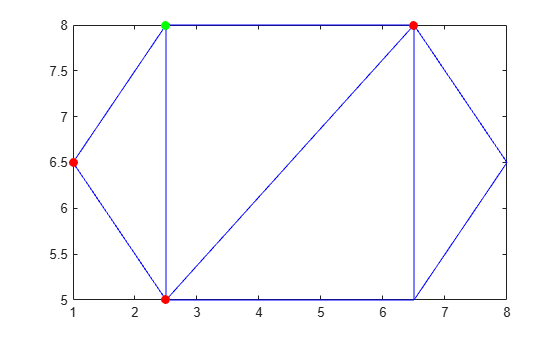edges

Triangulation edges

Description

example

E = edges(TR) returns the triangulation edges as a two-column matrix of vertex identification numbers. Vertex identifications are the row numbers of the triangulation vertices in TR.Points. The first column of E contains the starting vertex identification of each edge, and the second column contains the ending vertex identification.

Examples

collapse all

Load 2-D triangulation data and create a triangulation representation.

P = [2.5 8.0; 6.5 8.0; 2.5 5.0; 6.5 5.0; 1.0 6.5; 8.0 6.5];
T = [5 3 1; 3 2 1; 3 4 2; 4 6 2];
TR = triangulation(T,P);

Find the starting and ending vertex identification numbers of the edges in the triangulation.

E = edges(TR)
E = 9×2

1     2
1     3
1     5
2     3
2     4
2     6
3     4
3     5
4     6

Define the coordinates of the first point in the triangulation. Then define the coordinates of the three vertices sharing an edge with the first point (the second, third, and fifth).

startVert = TR.Points(1,:);
endVert = TR.Points([2 3 5],:);

Plot the triangulation. Plot the first vertex in green, and plot the connecting points along an edge in red.

triplot(TR)
hold on
plot(startVert(1,1),startVert(1,2),'g.','MarkerSize',20)
plot(endVert(:,1),endVert(:,2),'r.','MarkerSize',20)
hold offInput Arguments

collapse all

Triangulation representation, specified as a scalar triangulation or delaunayTriangulation object.

Data Types: triangulation | delaunayTriangulation